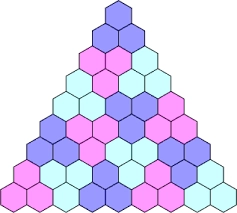# Arithmetic 81808

An increasing arithmetic sequence has an odd number of terms. The middle term is 302. If we remove the 4 largest terms from the sequence, the middle term will be 296. Determine the difference in the sequence.

d =  3

### Step-by-step explanation:Did you find an error or inaccuracy? Feel free to write us. Thank you!

Tips for related online calculators
Do you have a linear equation or system of equations and looking for its solution? Or do you have a quadratic equation?